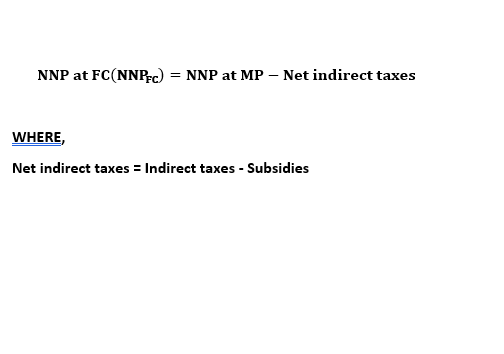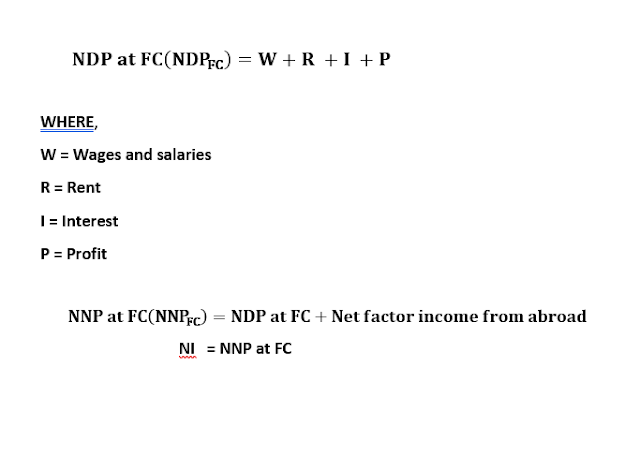# Concepts of Net National Product (NNP) | National Income(NI) | NNP at Market Price and Factor Cost

0

NET NATIONAL PRODUCT (NNP)

Gross national product minus depreciation is called net national product. In other words, the net national product is the market value of all final goods and services after allowing for depreciation. It is also called national income at market price. When charges for depreciation are deducted from the gross national product, we get it. While producing goods and services, the machinery and other fixed capitals wear and tear. It is known as depreciation. By deducting the value of depreciation from the value of the gross national product in a year, we get the value of the net national product.

Thus,

NNP = GNP –  Depreciation

NNP at Market Price and Factor Cost

NNP measured at the actual market price is called NNP at market price whereas NNP measured as the sum of price paid to all factors of production in form of wages, profits, interest, and rent for their contribution in production is called NNP at factor cost. In order to calculate NNP at factor cost, we deduct net indirect taxes from NNP at market price. Net indirect tax is equal to indirect taxes minus subsidies.NATIONAL INCOME (NI)

National income is the total sum of earning of all factors of production in the form of wages, profits, rent, and interest plus net factor income from abroad. In other words, national income means the sum of all incomes earned by domestically owned factors of production for their contribution to the production of goods and services. There are four factors in production: land, labor, capital, and organization. These factors of production receive factor reward in the form of rent, wages, interest, and profit respectively. The sum of these factor rewards earned within-country gives NDP at factor cost. When we sum up net factor income from abroad to NDP at FC, we get NNP at factor cost which is the NI. The different stages of calculating NI are as follows:The above method is the factor income method. But in the product method, first of all, GDP at MP is calculated by the sum of the market value of all final goods and services produced within the domestic territory of the country. Thereafter, GNP at MP is calculated by adding net factor income from abroad, which is converted to the net national product at factor cost by deducting indirect taxes and summing up subsidies. NNP at FC is the national income. Therefore, national income is also known as the national income at factor cost, which means the sum of all incomes earned by resources suppliers for their contribution of land, labor, capital, and organizational ability, which go into the year’s net production. Hence, the sum of the income received by factors of production in the form of rent, wages, interest, and profit is called national income.

By product method, NI is calculated as follows: